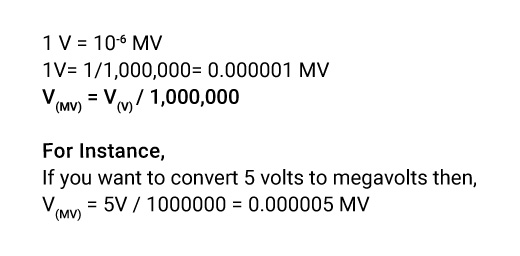## About Our V to MV Converter

“Conversion is a daily thing.”

Conversion is necessary but sometimes confusing. One wrong application of zero while converting can spoil the entire calculation. So, to ensure you at every step during calculations, we present a handy converter that will give you a quicker and more accurate result.

Whether you want to switch from volts to megavolts or another metric conversion, you can use our converter.

### How to Convert Volts to Megavolts?

You must divide the Voltage by the conversion ratio if you want to have volts to megavolt conversion. One megavolt is equal to one million volts. The Voltage in a megavolt is equal to the volts divided by 1,000,000.

### The Volts to Megavolts Conversion Formula.

You can use a direct formula for volts to megavolts conversion,If you don’t want to use a Volts to megavolts formula to convert volts to megavolts and still looking for a direct answer, then welcome to use our quick converter.

### How to Use Our Volts to Megavolts Converter?

Use our volts to megavolts converter in three simple steps,

• Step1: Click on our website to access our tool.
• Step2: Introduce the voltage value you want to convert.
• Step3: Press “convert” to switch to megavolts.

You can use this repeatedly to convert any voltage value to megavolts.

### How Many Volts Are in a Megavolt?

1MV = 106 V

1MV= 1,000,000V

One megavolt is equal to one million volts.

### What is the Difference Between Volts & Megavolts?

• Volt is the basic unit of Voltage, whereas it is a multiple unit of Voltage.
• Volt is the SI-derived unit, while megavolts are used in large quantities.
• V is the symbol of Voltage, and MV is the symbol of Megavolts.
• One volt is one millionth of a megavolt, and one megavolt equals one million volts.

### Examples of Converting Volts to Megavolts.

Though you have volts to megavolts calculator, have a quick view of the examples for a better understanding of the concept.

V(MV) = 2V / 1000000 = 0.000002 MV

V(MV) = 6V / 1000000 = 0.000006 MV

V(MV) = 8V / 1000000 = 0.000008 MV

V(MV) = 10V / 1000000 = 0.00001 MV

### Volt to Megavolts Conversion Table.

Just flip through the volts to the megavolts conversion table to enlighten with the pattern.

Volts Megavolts
1V 0.000001 MV
10V 0.00001MV
100V 0.0001MV
1,000V 0.001MV
10,000V 0.01MV
100,000V 0.1MV
1,000,000V 1MV